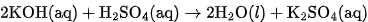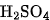×
Get Full Access to Introductory Chemistry - 5 Edition - Chapter 13 - Problem 90p
Get Full Access to Introductory Chemistry - 5 Edition - Chapter 13 - Problem 90p

×

# Solved: Determine the volume of 0.225 M KOH solution required to neutralize each sampleISBN: 9780321910295 34

## Solution for problem 90P Chapter 13

Introductory Chemistry | 5th Edition

• Textbook Solutions
• 2901 Step-by-step solutions solved by professors and subject experts
• Get 24/7 help from StudySoup virtual teaching assistantsIntroductory Chemistry | 5th Edition

4 5 1 289 Reviews
15
4
Problem 90P

Determine the volume of 0.225 M KOH solution required to neutralize each sample of sulfuric acid. The neutralization reaction is:

$$\mathrm {H_2SO_4}(aq)+2~\mathrm {KOH}(aq) \longrightarrow \mathrm{K_2SO_4}(aq)+2~\mathrm{H_2O}(l)$$

(a) 45 mL of 0.225 M $$\mathrm {H_2SO_4}$$

(b) 185 mL of 0.125 M $$\mathrm {H_2SO_4}$$

(c) 75 mL of 0.100 M $$\mathrm {H_2SO_4}$$

Equation Transcription:Text Transcription:

H_2SO_4(aq)+2 KOH(aq) rightarrow K_2SO_4(aq)+2 H_2O(l)

H_2SO_4

Step-by-Step Solution:

Step 1 of 8

Here, we are going to calculate the volume of KOH solution required to neutralize the given H2SO4 solutions.

Neutralisation reaction is the reaction between an acid and a base to give salt and water. The neutralization reaction in the given problem is:Here, 1 mole ofrequires 2 moles of KOH for complete neutralization.

___________________________

Step 2 of 8

Step 3 of 8

##### ISBN: 9780321910295

The full step-by-step solution to problem: 90P from chapter: 13 was answered by , our top Chemistry solution expert on 05/06/17, 06:45PM. Introductory Chemistry was written by and is associated to the ISBN: 9780321910295. This full solution covers the following key subjects: koh, reaction, acid, neutralization, neutralize. This expansive textbook survival guide covers 19 chapters, and 2046 solutions. Since the solution to 90P from 13 chapter was answered, more than 5837 students have viewed the full step-by-step answer. This textbook survival guide was created for the textbook: Introductory Chemistry, edition: 5. The answer to “?Determine the volume of 0.225 M KOH solution required to neutralize each sample of sulfuric acid. The neutralization reaction is: $$\mathrm {H_2SO_4}(aq)+2~\mathrm {KOH}(aq) \longrightarrow \mathrm{K_2SO_4}(aq)+2~\mathrm{H_2O}(l)$$(a) 45 mL of 0.225 M $$\mathrm {H_2SO_4}$$(b) 185 mL of 0.125 M $$\mathrm {H_2SO_4}$$(c) 75 mL of 0.100 M $$\mathrm {H_2SO_4}$$Equation Transcription:Text Transcription:H_2SO_4(aq)+2 KOH(aq) rightarrow K_2SO_4(aq)+2 H_2O(l)H_2SO_4” is broken down into a number of easy to follow steps, and 52 words.

Unlock Textbook Solution